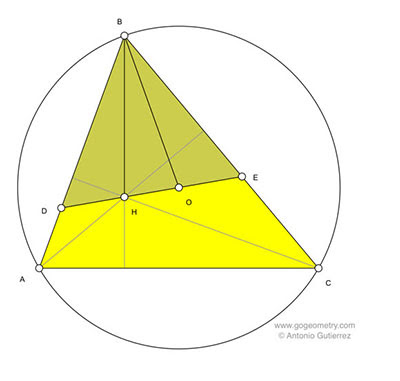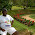## Thursday, August 27, 2015

### Geometry Problem 1147: Scalene Triangle, Orthocenter, Circumcenter, Congruence, Equilateral Triangle

Geometry Problem. Post your solution in the comments box below.
Level: Mathematics Education, High School, Honors Geometry, College.

Click the diagram below for more details.#### 3 comments:

1.By problem 778, BH² + AC² = 4R² or R² + AC² = 4R² which gives AC =√3*R. Moreover, AC/sin(B) =2R or sin(B)=AC/(2R) =√3*R/(2R)=√3/2 which implies that /_B = 60° ------(1)
Now refer to problem 1062. OH is the Euler line of a triangle with vertex /_B = 60°. Hence, CE-AD=OH,
BC-AB=OH. This further means, CE - AD = BC - AB or AB - AD = BC - CE or BD = BE --------(2)
(1) & (2) together imply that Tr. BDE is equilateral.

2.Let AO meet the circumcircle at N. Now <HCB =90-B= <NCB and hence HC//BN. But BH//NC, so BHCN is a //ogram and BH = NC = BO =ON =OC. Hence Tr. ONC is equilateral and so <B = 60.
So < AHC =120 = <AOC and hence AHOC is cyclic.
Also < CAO =30 =<CHO. Hence <BDE =60. So Tr. BDE has 2 angles = to 60 and the same is therefore equilateral.

Sumith Peiris
Moratuwa
Sri Lanka

3.Pl draw a figure if necessary
It is well-known that BH = 2.OX where OX is perp bisector of AC
and that OX = R cos B
So 2R cos B = BH = R implies B = 60 deg
Drop BF perpendicular to HO and extend it to meet the circle O at K.
Easy to see that BHKO is a rhombus
Equal arcs AK, CK subtend equal angles at B (each 30 deg)
BK perp to DE implies each of the angles BDE, BED = 60 deg
Hence triangle BDE is equilateral